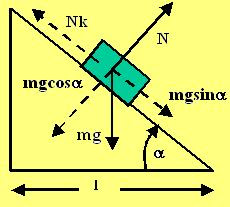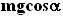## Tuesday, August 7, 2007

### Irodov Problem 1.66The force diagram of the body on the inclined plane is depicted in the figure beside. We shall resolve the forces acting on the mass along parallel and perpendicular directions to the slope of the inclined plane. Let the mass of the body be m.

Along the direction perpendicular to the mass there are two forces acting - i) the normal reaction from the surface N and ii) the component of gravity acting along the perpendicular direction. There is no component of acceleration along this direction and so we have,Along the direction parallel to the inclined plane there are two forces acting, i) the component of gravity along the parallel directionand ii) the force of friction that opposes the motion of the mass Nk. If w is the acceleration of the mass then, the dynamics of the mass is given by,The total length traveled by the mass can be determined by elementary trigonometry as.
Let the total time taken by the mass to reach the base be t. Then,Thus, t is minimized whenis maximized. The condition for extrema (maxima or minima) is given by,To ascertain whether indeed this is the maxima we must check that the second derivative is negative. The second derivative is given by,Now from (5) it is clear thatand in this intervalis positive. Thus, the second derivative ofis negative. Thus (5) corresponds to the minima.